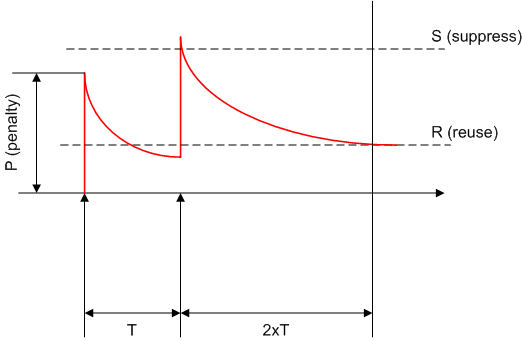blog
Optimizing IP Event Dampe ...
04 May 10

## Optimizing IP Event Dampening

Posted byPetr LapukhovThe purpose of event dampening is reducing the effect of oscillations on routing systems. In general, periodic process that affect the routing system as a whole should have the period no shorter than the system convergence time (relaxation time). Otherwise, the system will never stabilize and will be constantly updating its state. In reality, complex system have multiple periodic processes running at the same time, which results is in harmonic process interference and complex process spectrum. Considering such behavior is outside the scope of this paper. What we want to do, is finding optimal settings to filter high-frequency events from the routing system. In our particular case, events are interface flaps, occurring periodically. We want to make sure that oscillations with period T or less are not reported to the routing system. Here T is found empirically, based on observed/estimated convergence time as suggested above.

Event dampening uses exponential back-off algorithm to suppress event reporting to the upper level protocols. Effectively, every time an interface flaps (goes down, to be accurate) a penalty value of P is added to the interface penalty counter. If at some point the accumulated penalty exceeds the "suppress" value of S, the interface is placed in the suppress state and further link events are not reported to the upper protocol modules. At all time, the interface penalty counter follows exponential decay process based on the formula P(t)=P(0)*2^(-t/H) where H is half-life time setting for the process. As soon as accumulated penalty reaches the lower boundary of R - the reuse value, interface is unsuppressed, and further changes are again reported to the upper level protocols.What we want to find out, is the lowest value of H that suppresses all harmonic oscillation processes with the period of T or lower, but does not suppress longer-period processes. E.g. we want to block all oscillations happening every 5 seconds or more often, but report interface flaps happening every 6 seconds or less often. Look at the figure above and consider that there have been two flap events, separated by time period T. At the moment of the second flap, the suppress condition is: P*2^(-T/H)+P >= S. Here the left part is the penalty accumulated at the moment of the second flap, assuming the initial penalty at the moment of the first flap was zero. From this inequality, we quickly find out that H >= T/log2(P/(S-P)). if we could make P/(S-P)=2, then the formula would be greatly simplified. Per Cisco's implementation, P (penalty) is fixed to 1000, and by setting S=1500 we get 1000/(1500-1000)=2. Therefore, if we select S=1500, P=1000 then our condition becomes H >= T. Since we are looking for the minimal value of H we can set H=T. Seeded with this values, event dampening filter will reject all oscillating porcesses with the period shorter than T. However, there is one more parameter we are left to find is R - the reuse time.

We may apply the following logic here. Observing no further events since the last flap for the duration of 2xT, we may assume that the periodic process has stopped. Therefore, we may unblock the interface after 2xT seconds. The reuse value could be found by taking the penalty accumulated after the second flap, and further decaying it for 2xT more seconds: (P*2^(-T/H)+P)*2^(-2T/H) <= R. Since we set H=T we quickly find out that R >= 3/8*P = 375. At this point we have all parameters we need to know in order to apply optimal event dampening settings based on the cut-off period for oscillating processes. Here is a sample configuration, for T=10 seconds. Notice the last parameter, know as the maximum suppress time - the maximum time that the interface could be kept in suppress state. Since our goal is to hold the interface suppressed for at least 2xT seconds, the maximum suppress time is twice the half-life value.

```R3:
interface FastEthernet 0/0
dampening  10 375 1500 20
```

Lastly, a few words on figuring out the convergence time for your network. To being with, we only consider IGP protocols in this discussion. Dampening in BGP is more complicated, due to the scale of the routing system involved. The general consensus nowadays is that using dampening in BGP may result in more harm than good, due to cascading withdrawn messages. Next, for the IGPs, you are generally considered with a single fault domain, which in properly designed network is bounded to one IGP area (or EIGRP query scope zone). Convergence time for a single area depends on the following factors:

• Area size - impacts routing database sizes, affects LSA/Query propagation time and SPF runtime.
• Weakest (in terms of CPU/Memory) router in the area - this is the router to complete SPF computations the last.
• RIB/FIB sizes: a significant amount of time is wasted on updating RIB/FIB tables after IGP re-convergence. Again, depends on the area size

To summarize, the main factor is the area size and the number of links in the area (which normally follows the power law based on the number of nodes). However, knowing this fact does not give us a formula for the convergence time. In most cases, you should rely on empirical evidence to obtain this. Starting with one-two seconds could be reasonable, but you should scale this value by the factor of two or three to account for multiple oscillations that may run in the network concurrently. Still, one again, there is no magical formula for this - this is what network engineers and designers are for!